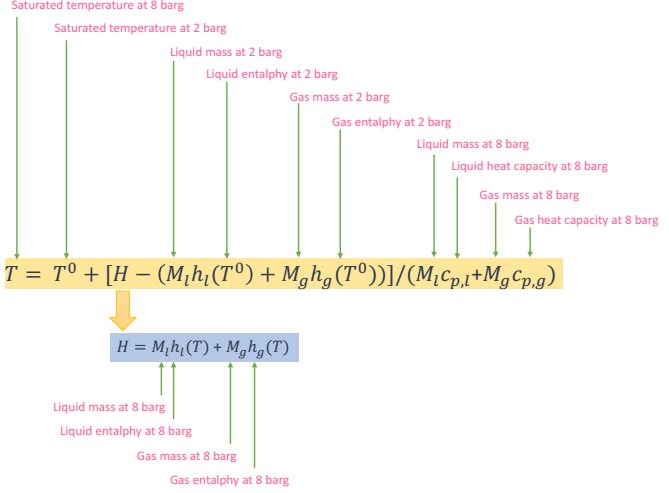# Predicting Holding Time of LNG in Storage Tank Without Venting

In this post, we use vacuum insulated type LNG storage tank when we don’t want to deal with boil-off gas handling. The storage tank have design pressure as high as 6-10 barg. When we store LNG in the LNG, boil-off gas will be generated due to heat leak to tank. Increasing temperature of LNG will increase total volume of LNG and vapor in the tank, thus increasing its pressure.

This phenomena leads to a simple question, “How long can LNG storage tank keep the vapor before PSV works?”

I have browsed about this and found this paper is very useful to predict holding time of LNG in storage tank without venting. I tried to do reverse engineering from the paper, but I confused… and failed.

But, the good news is I found other method to predict the holding time. However, I need you (whoever have experience in this area) to check if this method is right. I adopted the calculation from the paper and with iteration I found that my calculation is not much different from what vendor did.

From vendor information I got that: for LNG storage tank 1000 m3 with evaporation rate 0.07%/day, LNG can hold vapor from 2 barg to 8 barg for 135 days (at certain mixing value).

Step 1: Prepare the data

Prepare the data at 2 barg and 8 barg of LNG. The data required is :

• Saturated Pressure
• Temperature
• Density of liquid
• Density of gas
• Mass entalphy of liquid
• Mass entalphy of vapor
• Mass heat capacity of liquid
• Mass heat capacity of gas

I used LNG composition B from this post.

I prepare several data for different pressure to check if my calculation is correct at different condition.

Step 2: Use this equation to check the holding time

This is iterative part of this process.The data we need to calculate first is :

Mass of liquid at 2 barg = effective volume of liquid at tank (m3) x liquid density of 2 barg (kg/m3)

Mass of gas at 2 barg = assuming zero

The data we need to assume is :

Mass of liquid at 8 barg = Initial mass – mass of gas at 8 barg

Mass of gas at 8 barg = LNG evaporation rate x initial mass x day of holding time

We need to assume day of holding time.

FYI, initial mass is = mass of liquid at 2 barg + mass of gas at 2 barg. This mass is always the same regardless of pressure at temperature. So total mass at 2 barg is the same as total mass at 8 barg. But, total volume at 2 barg is lower than total volume of 8 barg.

By using equation above we will get “calculated T” . You will get calculated T value which is different from actual T (T at 8 barg which is -124.27 deg C). By using Microsoft Excel, subtract “calculated T” and “actual T”. By using Goal Seek in Microsoft Excel, set difference between “calculated T” and “actual T” to zero by changing holding time. Tada! You get estimated holding time of LNG at 1000 m3 from 2 barg to 8 barg. I got 145 days holding time.

My result is 7% different from the data from vendor. So, to make it more conservative, maybe we need to subtract 5-10% from calculated result.

Important note:

I tried to check if this method is actually right. I used other case. I used the data from Chart isotank to predict holding time.

By using 20 ft isotank with LNG evaporation rate of 0.25%/day, holding time is 52 days for isotank wigh design pressure 0f 10 barg. I found unreasonable holding time. I think it must be clear what initial pressure is. When initial pressure is 0, 2, and 6 barg, I found holding time is 133 days, 103 days, and 80 days, respectively. I tried to simulate if initial pressure is 8 barg, but holding time is not clear (the value is minus). So, I recommend you to better ask vendor when dealing with holding time. Predicting by yourself is good, but only vendor who can guarantee the performance of their tanks.